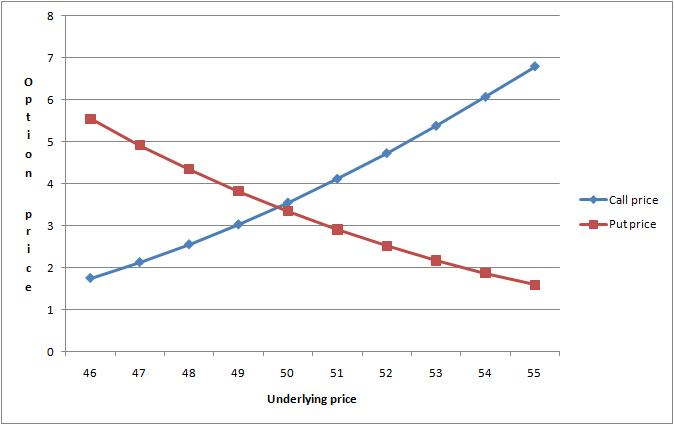# Option Pricing With the Black-Scholes-Merton Model

Options are financial contracts between two parties that give the buyer the right (and not the obligation) to buy (call option) or sell (put option) a particular asset (stock, index, futures, commodity, currency, interest rate, etc…) that is called underlying at a specified price (the strike price) and at a specified time (the expiration date). If the option can be exercised any time before expiration, it is called American option, if the option can be exercised only on the expiration date it is called European option.

To estimate the value of an option several different methods exist. One of the simplest methods to evaluate the price of an option is the Black-Scholes-Merton (BSM) model that has been published in the 1970s. Merton and Scholes eventually received a Nobel Prize in economics for their research on this subject. The BSM model assumes that the price follows a Geometric Brownian Motion where the price distribution is Gaussian and the interest rate is constant. These simplifications are not always acceptable in real world markets that are far from being Gaussian, where interest rates are not constant and where volatility is not constant. Nonetheless option pricing based on BSM is widely used in practice because of its simplicity and clearness.

The BSM model is the solution of the following Partial Differential Equation:

Where V is the price of the option, t is the time, σ is the volatility of the underlying, S is the price of the underlying, r is the risk-free interest rate. Starting from the above partial differential equation, a number of quantities have been derived with the intent to analyze the variation of option quantities with respect to changes in the underlying parameters.

## Option Greeks

Delta $\Delta$ measures the change of the price of the option with respect to a change in the price of the underlying:

Gamma $\Gamma$ measures the change of delta with respect to a change in the price of the underlying:

Vega measures the change of the price of the option with respect to a change in volatility:

Theta $\theta$ measures the change of the price of the option with respect to time:

Rho ρ measures the change of the price of the option with respect to a change in the interest rate:

This is an example of the pricing of a call and put option:The BSM model can be used to compute the implied volatility of an option using the known option price, underlying price, strike price, time to maturity and interest rate of the same option at different maturities and strike prices. The computation gives shape to the well-known volatility smile that can be observed almost on any underlying and reflects the probability of extreme moves. The volatility smile shows lower implied volatility in the at-the-money options and higher volatility in the in-the-money or out-of-the-money options.

The example below shows a typical volatility smile computed from market data:You can download the following example of Options Pricing built using the methods available in Ipredict’s library, the example contains also a worksheet with an example Delta Neutral Strategy applied to a hypothetical stock.

 Forecasting Methods Holt Winter’s, Series Decomposition and Wavelet Benchmarks Time Series Forecasting Use of the Moving Average in Time-series Forecasting Forecasting Concepts Denoising Techniques Error Statistics Computational Performance Fast Fourier Transform Moving Averages Kernel Smoothing Active Moving Average Savitsky-Golay Smoothing Fractal Projection Downloading Financial Data from Yahoo Multiple Regression Digital Signal Processing Principal Component Analysis Curve Analysis Options Pricing with Black-Scholes Markowitz Optimal Portfolio Time-series preprocessing